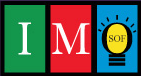### Unlimited Printable Worksheets, Online Practice and Online Tests For IMOInternational Mathematics Olympiad is an annual exam conducted by Science Olympiad Foundation. The IMO is conducted on two levels each year. Students from class I-XII may participate in the examination.

• 5th December 2019
• | 17th December 2019

### Class 1

Numbers, Addition, Subtraction, Shapes, Time, Money, Measurement, Data Handling, Patterns, Logical Reasoning

### Class 2

Numbers, Addition and Subtraction, Measurement, Patterns, Shapes, Time, Data Handling, Logical Reasoning

### Class 3

Numbers, Computation Operations, Fractions, Money, Measurement, Geometry, Data Handling, Logical Reasoning

### Class 4

Numbers, Arithmetic Operations, Factors and Multiples, Symmetry and Patterns, Fractions and Decimals, Measurement, Geometry, Money, Area and Perimeter, Time, Data Handling, Logical Reasoning

### Class 5

Arithmetic Operations, Simplification of Number Sentences, Factors and Multiples, Fractions and Decimals, Measurement, Percentage, Time, Money, Geometry, Area, Perimeter and Volume, Data Handling, Logical Reasoning

### Class 6

Numbers, Integers, Fractions and Decimals, Algebra, Mensuration, Geometry, Ratio and Proportion, Symmetry, Data Handling, Logical Reasoning

### Class 7

Integers, Fractions and Decimals, Algebra, Percentage, Simple Interest, Geometry, Perimeter and Area, Triangles, Exponents and Powers, Symmetry, Data Handling, Logical Reasoning

### Class 8

Rational Numbers, Direct and Inverse Proportions, Percentage, Simple and Compound Interest, Squares and Square Roots, Cubes and Cube Roots, Algebra, Mensuration, Geometry, Exponents and Powers, Data Handling, Logical Reasoning

### Class 9

Number System, Polynomials, Coordinate Geometry, Linear Equations, Triangles and Quadrilaterals, Lines and Angles, Circles, Mensuration, Statistics, Probability, Logical Reasoning

### Class 10

Real Numbers, Polynomials, Linear Equations, Quadratic Equations, Arithmetic Progressions, Triangles, Coordinate Geometry, Trigonometry, Circle, Mensuration, Statistics, Probability, Logical Reasoning